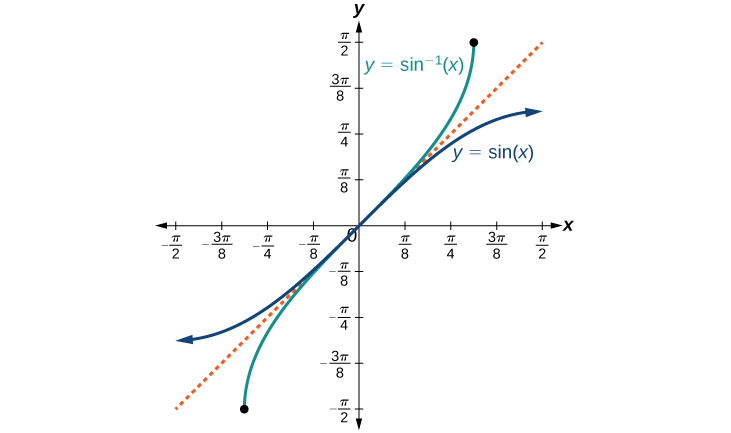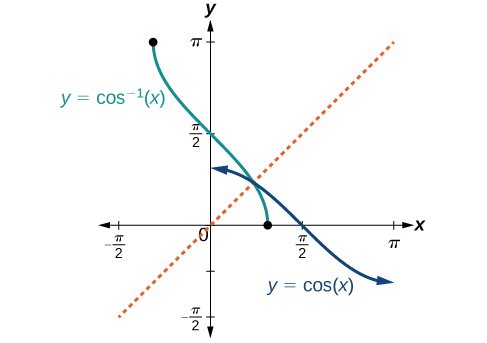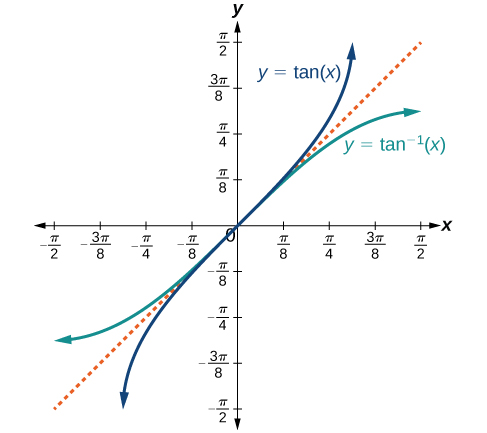# 8.3 Inverse trigonometric functions  (Page 2/15)

 Page 2 / 15

On these restricted domains, we can define the inverse trigonometric functions .

• The inverse sine function     $\text{\hspace{0.17em}}y={\mathrm{sin}}^{-1}x\text{\hspace{0.17em}}$ means $\text{\hspace{0.17em}}x=\mathrm{sin}\text{\hspace{0.17em}}y.\text{\hspace{0.17em}}$ The inverse sine function is sometimes called the arcsine    function, and notated $\text{\hspace{0.17em}}\mathrm{arcsin}x.$
• The inverse cosine function     $\text{\hspace{0.17em}}y={\mathrm{cos}}^{-1}x\text{\hspace{0.17em}}$ means $\text{\hspace{0.17em}}x=\mathrm{cos}\text{\hspace{0.17em}}y.\text{\hspace{0.17em}}$ The inverse cosine function is sometimes called the arccosine    function, and notated $\text{\hspace{0.17em}}\mathrm{arccos}\text{\hspace{0.17em}}x.$
• The inverse tangent function     $\text{\hspace{0.17em}}y={\mathrm{tan}}^{-1}x\text{\hspace{0.17em}}$ means $\text{\hspace{0.17em}}x=\mathrm{tan}\text{\hspace{0.17em}}y.\text{\hspace{0.17em}}$ The inverse tangent function is sometimes called the arctangent    function, and notated $\text{\hspace{0.17em}}\mathrm{arctan}\text{\hspace{0.17em}}x.$

The graphs of the inverse functions are shown in [link] , [link] , and [link] . Notice that the output of each of these inverse functions is a number, an angle in radian measure. We see that $\text{\hspace{0.17em}}{\mathrm{sin}}^{-1}x\text{\hspace{0.17em}}$ has domain $\text{\hspace{0.17em}}\left[-1,1\right]\text{\hspace{0.17em}}$ and range $\text{\hspace{0.17em}}\left[-\frac{\pi }{2},\frac{\pi }{2}\right],$ ${\mathrm{cos}}^{-1}x\text{\hspace{0.17em}}$ has domain $\text{\hspace{0.17em}}\left[-1,1\right]\text{\hspace{0.17em}}$ and range $\text{\hspace{0.17em}}\left[0,\pi \right],\text{\hspace{0.17em}}$ and $\text{\hspace{0.17em}}{\mathrm{tan}}^{-1}x\text{\hspace{0.17em}}$ has domain of all real numbers and range $\text{\hspace{0.17em}}\left(-\frac{\pi }{2},\frac{\pi }{2}\right).\text{\hspace{0.17em}}$ To find the domain    and range    of inverse trigonometric functions, switch the domain and range of the original functions. Each graph of the inverse trigonometric function is a reflection of the graph of the original function about the line $\text{\hspace{0.17em}}y=x.$The sine function and inverse sine (or arcsine) functionThe cosine function and inverse cosine (or arccosine) functionThe tangent function and inverse tangent (or arctangent) function

## Relations for inverse sine, cosine, and tangent functions

For angles in the interval $\text{\hspace{0.17em}}\left[-\frac{\pi }{2},\frac{\pi }{2}\right],\text{\hspace{0.17em}}$ if $\text{\hspace{0.17em}}\mathrm{sin}\text{\hspace{0.17em}}y=x,\text{\hspace{0.17em}}$ then $\text{\hspace{0.17em}}{\mathrm{sin}}^{-1}x=y.$

For angles in the interval $\text{\hspace{0.17em}}\left[0,\pi \right],\text{\hspace{0.17em}}$ if $\text{\hspace{0.17em}}\mathrm{cos}\text{\hspace{0.17em}}y=x,\text{\hspace{0.17em}}$ then $\text{\hspace{0.17em}}{\mathrm{cos}}^{-1}x=y.$

For angles in the interval $\text{\hspace{0.17em}}\left(-\frac{\pi }{2},\frac{\pi }{2}\right),\text{\hspace{0.17em}}$ if $\text{\hspace{0.17em}}\mathrm{tan}\text{\hspace{0.17em}}y=x,\text{\hspace{0.17em}}$ then $\text{\hspace{0.17em}}{\mathrm{tan}}^{-1}x=y.$

## Writing a relation for an inverse function

Given $\text{\hspace{0.17em}}\mathrm{sin}\left(\frac{5\pi }{12}\right)\approx 0.96593,\text{\hspace{0.17em}}$ write a relation involving the inverse sine.

Use the relation for the inverse sine. If $\text{\hspace{0.17em}}\mathrm{sin}\text{\hspace{0.17em}}y=x,\text{\hspace{0.17em}}$ then $\text{\hspace{0.17em}}{\mathrm{sin}}^{-1}x=y$ .

In this problem, $\text{\hspace{0.17em}}x=0.96593,\text{\hspace{0.17em}}$ and $\text{\hspace{0.17em}}y=\frac{5\pi }{12}.$

${\mathrm{sin}}^{-1}\left(0.96593\right)\approx \frac{5\pi }{12}$

Given $\text{\hspace{0.17em}}\mathrm{cos}\left(0.5\right)\approx 0.8776,$ write a relation involving the inverse cosine.

$\mathrm{arccos}\left(0.8776\right)\approx 0.5$

## Finding the exact value of expressions involving the inverse sine, cosine, and tangent functions

Now that we can identify inverse functions, we will learn to evaluate them. For most values in their domains, we must evaluate the inverse trigonometric functions by using a calculator, interpolating from a table, or using some other numerical technique. Just as we did with the original trigonometric functions, we can give exact values for the inverse functions when we are using the special angles, specifically $\text{\hspace{0.17em}}\frac{\pi }{6}\text{\hspace{0.17em}}$ (30°), $\text{\hspace{0.17em}}\frac{\pi }{4}\text{\hspace{0.17em}}$ (45°), and $\text{\hspace{0.17em}}\frac{\pi }{3}\text{\hspace{0.17em}}$ (60°), and their reflections into other quadrants.

Given a “special” input value, evaluate an inverse trigonometric function.

1. Find angle $\text{\hspace{0.17em}}x\text{\hspace{0.17em}}$ for which the original trigonometric function has an output equal to the given input for the inverse trigonometric function.
2. If $\text{\hspace{0.17em}}x\text{\hspace{0.17em}}$ is not in the defined range of the inverse, find another angle $\text{\hspace{0.17em}}y\text{\hspace{0.17em}}$ that is in the defined range and has the same sine, cosine, or tangent as $\text{\hspace{0.17em}}x,$ depending on which corresponds to the given inverse function.

## Evaluating inverse trigonometric functions for special input values

Evaluate each of the following.

1. ${\text{sin}}^{-1}\left(\frac{1}{2}\right)$
2. ${\text{sin}}^{-1}\left(-\frac{\sqrt{2}}{2}\right)$
3. ${\mathrm{cos}}^{-1}\left(-\frac{\sqrt{3}}{2}\right)$
4. ${\mathrm{tan}}^{-1}\left(1\right)$
1. Evaluating $\text{\hspace{0.17em}}{\mathrm{sin}}^{-1}\left(\frac{1}{2}\right)\text{\hspace{0.17em}}$ is the same as determining the angle that would have a sine value of $\text{\hspace{0.17em}}\frac{1}{2}.\text{\hspace{0.17em}}$ In other words, what angle $\text{\hspace{0.17em}}x\text{\hspace{0.17em}}$ would satisfy $\text{\hspace{0.17em}}\mathrm{sin}\left(x\right)=\frac{1}{2}?\text{\hspace{0.17em}}$ There are multiple values that would satisfy this relationship, such as $\text{\hspace{0.17em}}\frac{\pi }{6}\text{\hspace{0.17em}}$ and $\text{\hspace{0.17em}}\frac{5\pi }{6},\text{\hspace{0.17em}}$ but we know we need the angle in the interval $\text{\hspace{0.17em}}\left[-\frac{\pi }{2},\frac{\pi }{2}\right],\text{\hspace{0.17em}}$ so the answer will be $\text{\hspace{0.17em}}{\mathrm{sin}}^{-1}\left(\frac{1}{2}\right)=\frac{\pi }{6}.\text{\hspace{0.17em}}$ Remember that the inverse is a function, so for each input, we will get exactly one output.
2. To evaluate $\text{\hspace{0.17em}}{\mathrm{sin}}^{-1}\left(-\frac{\sqrt{2}}{2}\right),\text{\hspace{0.17em}}$ we know that $\text{\hspace{0.17em}}\frac{5\pi }{4}\text{\hspace{0.17em}}$ and $\text{\hspace{0.17em}}\frac{7\pi }{4}\text{\hspace{0.17em}}$ both have a sine value of $\text{\hspace{0.17em}}-\frac{\sqrt{2}}{2},\text{\hspace{0.17em}}$ but neither is in the interval $\text{\hspace{0.17em}}\left[-\frac{\pi }{2},\frac{\pi }{2}\right].\text{\hspace{0.17em}}$ For that, we need the negative angle coterminal with $\text{\hspace{0.17em}}\frac{7\pi }{4}:$ ${\text{sin}}^{-1}\left(-\frac{\sqrt{2}}{2}\right)=-\frac{\pi }{4}.\text{\hspace{0.17em}}$
3. To evaluate $\text{\hspace{0.17em}}{\mathrm{cos}}^{-1}\left(-\frac{\sqrt{3}}{2}\right),\text{\hspace{0.17em}}$ we are looking for an angle in the interval $\text{\hspace{0.17em}}\left[0,\pi \right]\text{\hspace{0.17em}}$ with a cosine value of $\text{\hspace{0.17em}}-\frac{\sqrt{3}}{2}.\text{\hspace{0.17em}}$ The angle that satisfies this is $\text{\hspace{0.17em}}{\mathrm{cos}}^{-1}\left(-\frac{\sqrt{3}}{2}\right)=\frac{5\pi }{6}.$
4. Evaluating $\text{\hspace{0.17em}}{\mathrm{tan}}^{-1}\left(1\right),\text{\hspace{0.17em}}$ we are looking for an angle in the interval $\text{\hspace{0.17em}}\left(-\frac{\pi }{2},\frac{\pi }{2}\right)\text{\hspace{0.17em}}$ with a tangent value of 1. The correct angle is $\text{\hspace{0.17em}}{\mathrm{tan}}^{-1}\left(1\right)=\frac{\pi }{4}.$

#### Questions & Answers

write down the polynomial function with root 1/3,2,-3 with solution
if A and B are subspaces of V prove that (A+B)/B=A/(A-B)
write down the value of each of the following in surd form a)cos(-65°) b)sin(-180°)c)tan(225°)d)tan(135°)
Prove that (sinA/1-cosA - 1-cosA/sinA) (cosA/1-sinA - 1-sinA/cosA) = 4
what is the answer to dividing negative index
In a triangle ABC prove that. (b+c)cosA+(c+a)cosB+(a+b)cisC=a+b+c.
give me the waec 2019 questions
the polar co-ordinate of the point (-1, -1)
prove the identites sin x ( 1+ tan x )+ cos x ( 1+ cot x )= sec x + cosec x
tanh`(x-iy) =A+iB, find A and B
B=Ai-itan(hx-hiy)
Rukmini
what is the addition of 101011 with 101010
If those numbers are binary, it's 1010101. If they are base 10, it's 202021.
Jack
extra power 4 minus 5 x cube + 7 x square minus 5 x + 1 equal to zero
the gradient function of a curve is 2x+4 and the curve passes through point (1,4) find the equation of the curve
1+cos²A/cos²A=2cosec²A-1
test for convergence the series 1+x/2+2!/9x3ByByByByByBy Mariah Hauptman